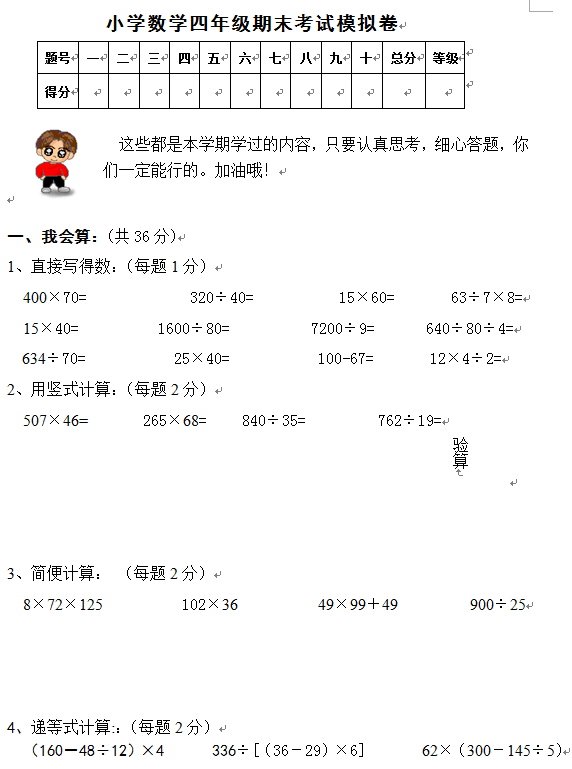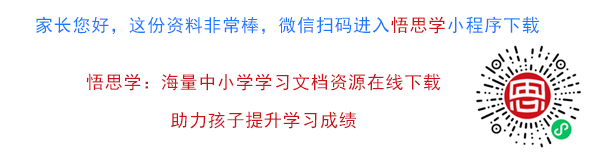1、直接写得数：（每题1分）

400×70=             320÷40=            15×60=        63÷7×8=

15×40=          1600÷80=           7200÷9=       640÷80÷4=

634÷70=           25×40=           100-67=       12×4÷2=

【DOC文档8页】小学人教版四年级上册数学期末试题2及参考答案A4电子版资料_可直接打印_会员免费下载##### 评论信息